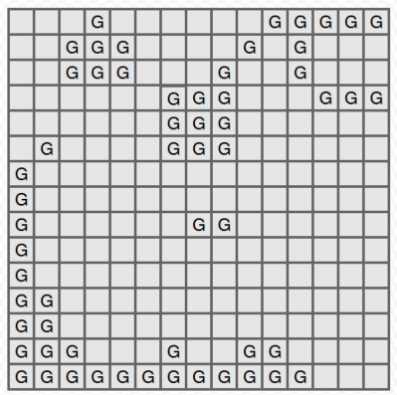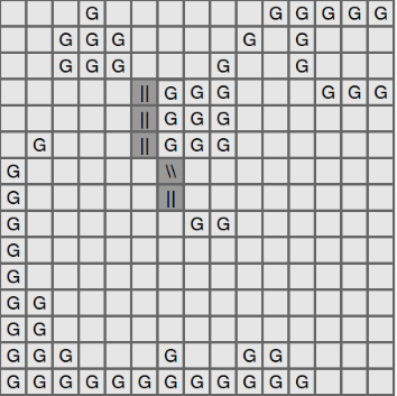# Gold MinesBUET CSE Day 2016 Program...
Limits 2s, 512 MB

The city of gridland is a grid with n rows and m columns. The city can be divided into n*m cells. A cell in is denoted by a coordinate (x, y) where x is the row number and y is the column number.

Two cells are adjacent if they share a side or a corner. A cell can have at most 8 adjacent cells. A path between two cells is defined by a sequence of adjacent nodes between the first cell and the last cell.

The scientists recently discovered gold in some of the cells. They marked the positions of all the cells which contain gold. Here is a sample map:In the figure above, all the cells marked “G” has gold in them. Let’s call the “gold-cells”.

Two gold-cells are part of the same goldmine if:

• There is a path between the cells
• All the cells which belong to the path contain gold.

In the figure above, there are 4 gold mines. Size of a gold mine is the number of gold cells in the mine. In the figure above, the top-left gold mine has size 7.

The government decided to select exactly two gold mines and connect them by a tunnel. A tunnel is a path between two cells. But to qualify as a tunnel, one end of the path must be adjacent to the first gold-mine, another end of the path must be adjacent to the second gold-mine. Also The path must not go through any gold-cells. (Note that a tunnel can have length 1)

$\text{The cost of building a tunnel} = \text{length of the tunnel} \times \text{size of the first gold mine} \times \text{size of the second gold mine}$

Size of a gold mine is the number of gold cells in the mine.The second figure shows the optimal way to build tunnel for this map. The cost is $5 \times 7 \times 2 = 70$.

Given the configuration of the grid, print the minimum cost.

## Input

The first line contains $T$ ($1 ≤ T ≤ 120$) which describes the number of test cases. First line of each test cases contains two integers, $n$ and $m$ ($1 ≤ n, m ≤ 40$). Each of the next $n$ lines contains $m$ characters denoting the grid.

## Output

For each case, print the case number and the minimum cost. Print -1 without the quotation marks, if there is less than two gold mines in the grid.

## Sample

InputOutput
2
12 12
......GGGGG.
......G.....
G.....G.....
GG....G.....
GG....G.....
G...G.GG....
.G..GGGG....
....GGGG....
.....G......
.......G....
............
............
5 5
GGGGG
G...G
G.G.G
G...G
GGGGG

Case 1: 21
Case 2: 16uDebug

### Submit